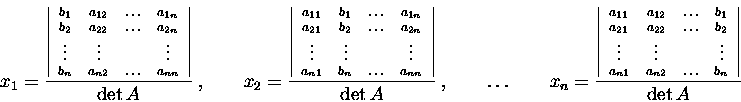LINEAR ALGEBRA (Math 211)
Sheet #4
DETERMINANTS 2

Transpose of a matrix.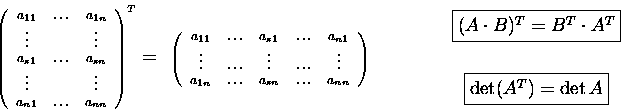Product and inverse.
Theorem. A matrix A is invertible if and only if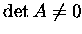.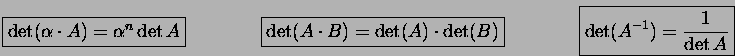Theorem. (Evaluating determinants by row reduction)
(1) If a row (or a column) of A is multiplied by a constant, thenis multiplied by.
(2) Interchanging any two rows (or columns) of A changes the sign of.
(3) If a multiple of one row (column) of A is added to another row (column) of A, then the determinant is unchanged.

Eigenvalues and eigenvectors
Definition. An eigenvalue of a matrix A is a value ofsuch that the matrix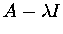is not invertible (roots of the characteristic equation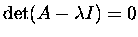).

Definition. An eigenvector corresponding to an eigenvalueof a matrix A is a nontrivial solution of the system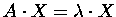Definition. Let A=(aij) be a square matrix. The minor Mij of an entry aij is the determinant of the matrix obtained from A by deleting the i-th row and j-th column. The number Cij=(-1)i+jMij is called the cofactor of antry aij.

Theorem. (Cofactor expansion)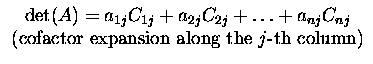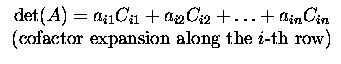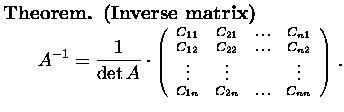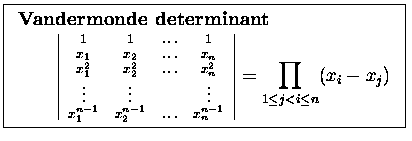Cramer's rule.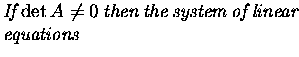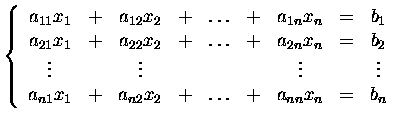has a unique solution